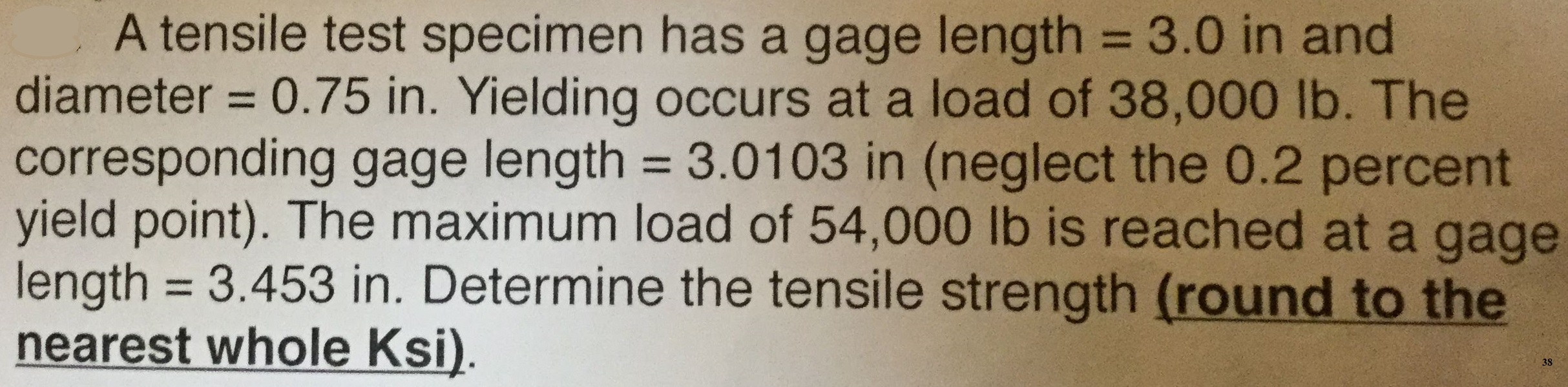# A tensile test specimen has a gage length = 3.0 in and diameter 0.75 in. Yielding occurs at a load of 38,000 lb. The corresponding gage length 3.01 03 in (neglect the 0.2 percent yield point). The maximum load of 54,000 lb is reached at a gage length 3.453 in. Determine the tensile strength (round to the nearest whole Ksi) 38

Questionhelp_outlineImage TranscriptioncloseA tensile test specimen has a gage length = 3.0 in and diameter 0.75 in. Yielding occurs at a load of 38,000 lb. The corresponding gage length 3.01 03 in (neglect the 0.2 percent yield point). The maximum load of 54,000 lb is reached at a gage length 3.453 in. Determine the tensile strength (round to the nearest whole Ksi) 38 fullscreen

### Want to see the step-by-step answer?